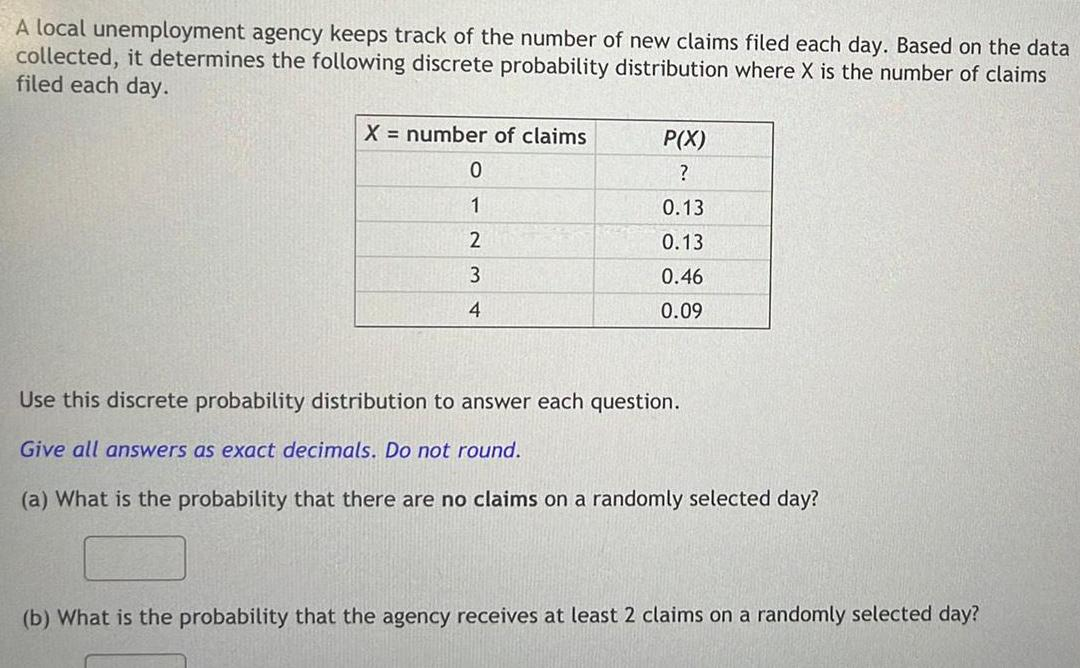Question:

# A local unemployment agency keeps track of the number of new

Last updated: 11/20/2023A local unemployment agency keeps track of the number of new claims filed each day Based on the data collected it determines the following discrete probability distribution where X is the number of claims filed each day X number of claims 0 1 2 3 4 P X 0 13 0 13 0 46 0 09 Use this discrete probability distribution to answer each question Give all answers as exact decimals Do not round a What is the probability that there are no claims on a randomly selected day b What is the probability that the agency receives at least 2 claims on a randomly selected day